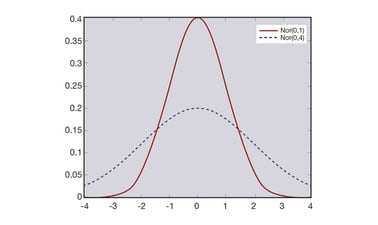# Probability and Statistics III: A Gentle Introduction to Statistics

This course provides an introduction to basic statistical concepts. We begin by walking through a library of probability distributions – including the normal distribution, which in turn leads to the Central Limit Theorem. We then discuss elementary descriptive statistics and estimation methods.This course is archived
Estimated 4 weeks
6–10 hours per week
Self-paced
Free

This course provides an introduction to basic statistical concepts.

We begin by walking through a library of probability distributions, where we motivate their uses and go over their fundamental properties.
These distributions include such important folks as the Bernoulli, binomial, geometric, Poisson, uniform, exponential, and normal distributions, just to name a few. Particular attention is paid to the normal distribution, because it leads to the Central Limit Theorem (the most-important mathematical result in the universe, actually), which enables us to make probability calculations for arbitrary averages and sums of random variables.

We then discuss elementary descriptive statistics and estimation methods, including unbiased estimation, maximum likelihood estimation, and the method of moments – you gotta love your MoM! Finally, we describe the t, χ2, and F sampling distributions, which will prove to be useful in upcoming statistical applications.

### At a glance

• Institution: GTx
• Subject: Data Analysis & Statistics
• Level: Intermediate
• Prerequisites:

Learners will be expected to come in knowing a bit of set theory and basic calculus, as well as the material from the first two courses in this series (the Gentle Introduction to Probability and Random Variables courses). The prerequisite material is all available for you to access; and in any event, we will try to make the current course as self-contained as possible. In addition, this course will involve a bit of computer programming, so it would be nice to have at least a little experience in something like Excel and/or the R freeware statistical package.

• Language: English

# What you'll learn

Skip What you'll learn

Upon completion of this course, learners will be able to:

• Review a library of discrete and continuous probability distributions
• Recognize normal distribution and the Central Limit Theorem, and how they are applied in practice
• Recognize elementary methods of descriptive statistics
• Describe methods that can be used to estimate the unknown parameters of a distribution
• Identify statistical sampling distributions

# Syllabus

Skip Syllabus

“FCPS” refers to the free text, A First Course in Probability and Statistics

Module 1: Distributions
• Lesson 1: Bernoulli and Binomial Distributions (FCPS §4.1.1)
• Lesson 2: Hypergeometric Distribution (FCPS §4.1.2)
• Lesson 3: Geometric and Negative Binomial Distributions (FCPS §4.1.3)
• Lesson 4: Poisson Distribution (FCPS §4.1.4)
• Lesson 5: Uniform, Exponential, and Friends (FCPS §4.2.1–4.2.2)
• Lesson 6: Other Continuous Distributions (FCPS §4.2.3)
• Lesson 7: Normal Distribution: Basics (FCPS §4.3.1)
Module 1 (cont’d): Distributions
• Lesson 8: Standard Normal Distribution (FCPS §4.3.2)
• Lesson 9: Sample Mean of Normals (FCPS §4.3.3)
• Lesson 10: The Central Limit Theorem + OPTIONAL Proof (FCPS §4.3.4)
• Lesson 11: Central Limit Theorem Examples (FCPS §4.3.5)
• Lesson 12 [OPTIONAL]: Extensions – Multivariate Normal Distribution (FCPS §4.4.1)
• Lesson 13 [OPTIONAL]: Extensions – Lognormal Distribution (FCPS §4.4.2)
• Lesson 14: Computer Stuff, including OPTIONAL Box-Muller Proof (FCPS §4.5)
Module 2: Getting Started with Statistics
• Lesson 1: Introduction to Descriptive Statistics (FCPS §5.1.1)
• Lesson 2: Summarizing Data (FCPS §5.1.2)
• Lesson 3: Candidate Distributions (FCPS §5.1.3)
• Lesson 4: Introduction to Estimation (FCPS §5.2.1)
• Lesson 5: Unbiased Estimation (FCPS §5.2.2)
• Lesson 6: Mean Squared Error (FCPS §5.2.3)
Module 2 (cont’d): Getting Started with Statistics
• Lesson 7: Maximum Likelihood Estimation (FCPS §5.2.4)
• Lesson 8: Trickier MLE Examples (FCPS §5.2.4)
• Lesson 9: Invariance Property of MLEs (FCPS §5.2.4)
• Lesson 10: Method of Moments Estimation (FCPS §5.2.5)
• Lesson 11: Sampling Distributions (FCPS §5.3)